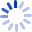# Mathematics: Queen and Servant of Science

An absorbing account of pure and applied mathematics from the geometry of Euclid to that of Riemann, and its application in Einstein's theory of relativity. The twenty chapters cover such topics as: algebra, number theory, logic, probability, infinite sets and the foundations of mathematics, rings, matrices, transformations, groups, geometry, and topology. Mathematics was republished in 1987 with corrections and an added foreword by Martin Gardner.

##### Cash for Textbooks